# MMoE论文笔记

### 摘要

• 多任务学习旨在构建单个模型来同时学习多个目标和任务。

• 但是，通常任务之间的关系会极大地影响多任务模型的预测质量。

Multi-gate Mixture-of-Experts(MMoE) 能够清晰地从数据中学习任务之间的关系：

• 将Mixture-of-Experts(MoE)的结构应用于多任务学习中
• 在所有任务共享expert submodels的同时，通过训练gating network来优化每一个任务。

• 实验表明在任务相关性比较弱的时候，MMoE会表现的更好。

### 一、引言

• 源于不同任务之间的固有的冲突事实上会对于至少一部分任务造成不利的影响，尤其当模型的参数在各个任务中被广泛共享的时候。

• 基于一个特定的数据生成假设前提去探究多任务学习中任务的区别，并且依据任务区别的程度去提出建议 -> 真实的数据具有极为复杂的模式，很难去明确地衡量任务之间的区别并依此去应用这些建议
• 也有不需要给出明确的任务区别程度的方法，但是为了适应不同的任务，往往带来更多的模型参数 -> 在资源有限的情况下，计算的成本是难以接受的

MMoE怎么样：

• MMoE对于任务之间的关系进行明确地建模，并且学习任务特定的函数去平衡共享的表达

MMoE explicitly models the task relationshipds and learns task-specific functionalities to leverage shared representations.

It allows parameters to be automatically allocated to capture either shared task information or task-specific inofrmation, avoiding the need of adding many new parameters per task.

MMoE结构概述：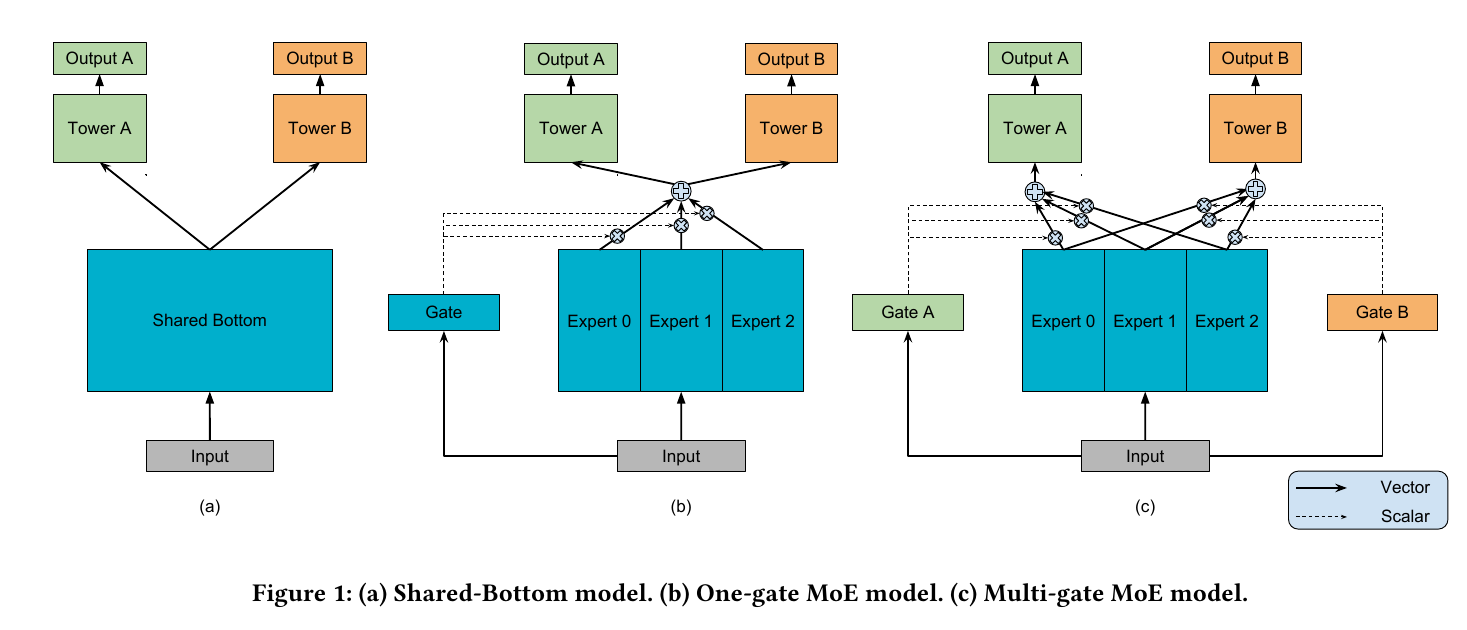• 基于Shared-Bottom multi-task DNN structure（如上图(a)）: Shared-Bottom: input -> bottom layers (shared) -> tower network (每个任务各自的)
• 如上图(c)，MMoE有一组bottom networks, 每一个叫做一个expert, 在本文中， expert network是一个feed-forward network
• 然后为每个任务引入一个gating network。Gating networks 的输入是input features， 输出是softmax gates，即各个expert的权重
• 这样的话，不同任务的gating networks能够学到不同的专家混合方式，以此捕捉到任务之间的关系
• MMoE更容易训练并且能够收敛到一个更好的loss，因为近来有研究发现modulation和gating机制能够提升训练非凸深度神经网络的可训练性。

2. 同时提升模型的表达力以及可训练性。

MMoE explicitly models task relationships. Through modulation and gating networks, our model autoatically ajusts parameterization between modeling shared information and modeling task-specific information.

MMoE improves both model expressiveness and trainability.

### 2.1 Multi-task Learning in DNNs

shared-bottom model struccture:

• 所有任务共享bottom hidden layers
• 减少过拟合的风险
• 但是会遭受由于任务的区分带来的优化冲突，因为所有任务在shared-bottom layers使用相同的参数集

• cross-stitch network, tensor factorization model

### 2.2 Ensemble of Subnets & Mixture of Experts

Eigen 和 Shazeer将mixture-of-experts model转变为基本的building blocks (MoE layer)，并且把它stack到一个DNN中：

• 在训练和测试的时候，MOE layer会依据这一层的输入来选择subnets(experts)

PathNet:

• 是一个巨大的神经网络，每一层都有multiple layers和multiple submodules

### 三、基本知识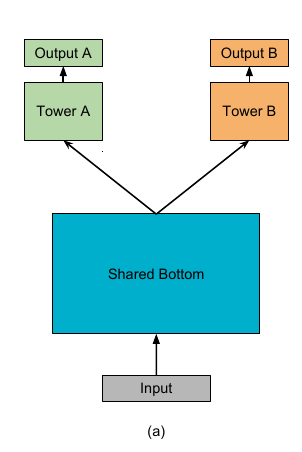• 给定$K$个任务，模型由一个shared-bottom network (由 $f$ 表达) 和 $K$ 个 tower networks $h^k$ 组成，其中 $k=1,2,\cdots,K$

• shared-bottom network跟在输入层后面，tower networks建立在shared-bottom 的输出之上，最后由每个tower产生各自任务的输出$y_k$

• 对于任务$k$，模型可以表达为：

$y_k=h^k(f(x))$

### 四、Modeling Approaches

#### 4.1 Mixture-of-Experts

$y=\sum_{i=1}^{n}g(x)_if_i(x)$

• $\sum_{i=1}^{n}g(x)_i=1$, $g(x)_i$ 表示expert $f_i$ 的权重
• $f_i, i=1,\cdots,n$$n$ 个expert networs
• 表示一个用于综合所有专家结果的gating network
• 换句话说，gating network $g$ 基于input产生$n$个experts上的一个分布，而最后的结果是所有experts输出的weighted sum。

MoE Layer:

• MoE layer和MOE model有着相同的结构，只不过是将前一层的输出作为输入，输出则会继续传到下一层。整个模型以端到端的方式训练
• MoE layer最开始提出的主要目的是进行conditional computation, 也就是说only parts of a network are active on a per-example basis
• 对于每个输入的example，模型能够通过gating network基于input选择a subset of experts。

#### 4.2 Multi-gate Mixture-of-Experts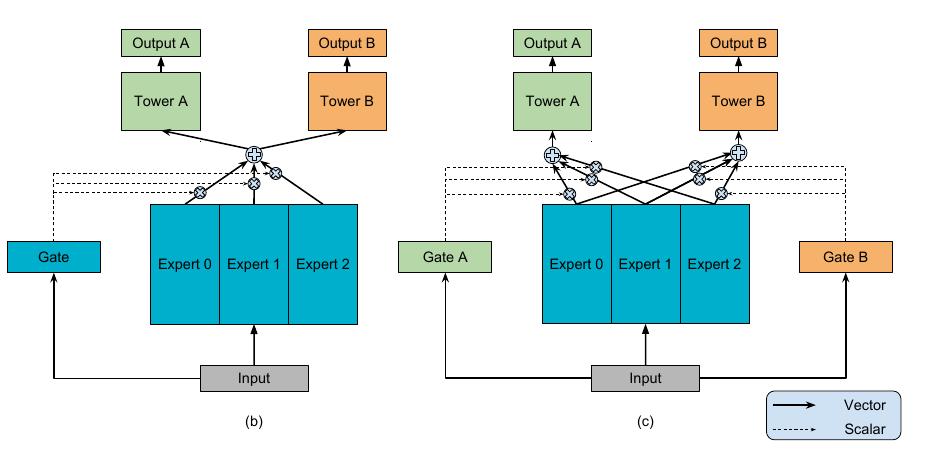• 本文提出一个新的MoE模型用于捕捉任务之间的区别，并且相对于shared-bottom多任务模型，本模型不会带来参数的极大增加
• 新的模型叫做Multi-gate Mixture-of-Experts(MMoE) model, 其主要思想是用MoE layer来替代share bottom network $f$
• 此外，对于每个任务$k$，都加入一个单独的gating network $g^k$
• 具体地，任务$k$的输出是：

$y_k = h^k(f^k(x))$

$f^k(x)=\sum_{i=1}^{n}g^k(x)_if_i(x)$

​ gating networks就是一个简单加上softmax layer的线性变换：

$g^k(x)=softmax(W_{gk}x)$

​ 其中$W_{gk}\in R^{n\times d}$$n$为experts的个数，$d$ 为特征维度。

github - Keras MMoE

• d: number of input features

• h: number of units per export

• n: number of experts

expert network: $W_{n\times h \times d}$

gating network: $W_{k\times n \times d}$

expert networks:

n个expert networks的输出$y\in R^{n\times h}$

$y=f(x)=W_{n\times h \times d}x + b_{k\times h} \quad \quad(i=1,\dots,n)$

k个gating networks的输出 $z \in R^{k\times n}$

$z= g(x)=softmax(W_{k\times n \times d}x + b_{k\times n})$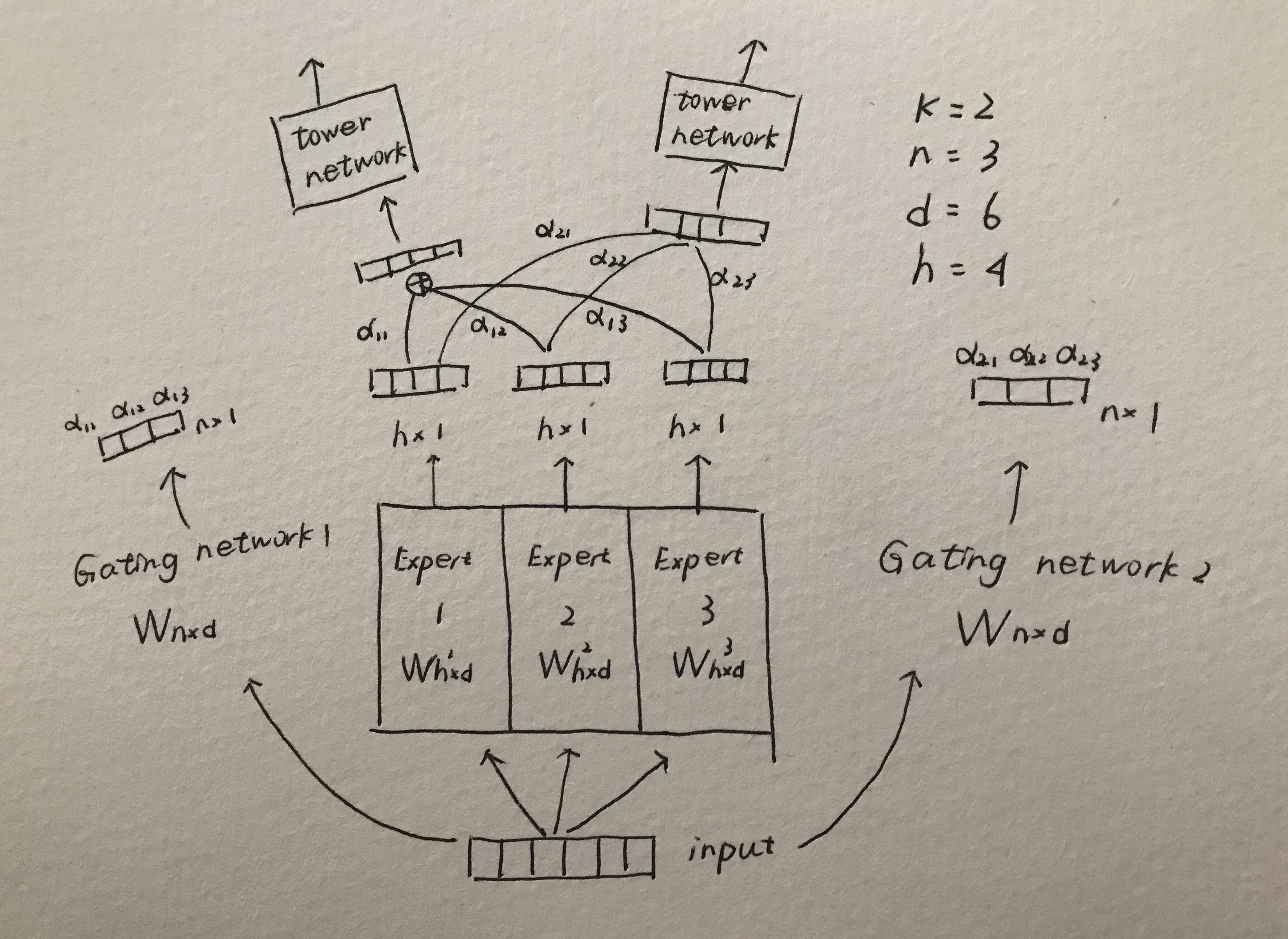### 五、MMoE on Synthetic Data

#### 5.1 Performance on Data with Different Task Correlations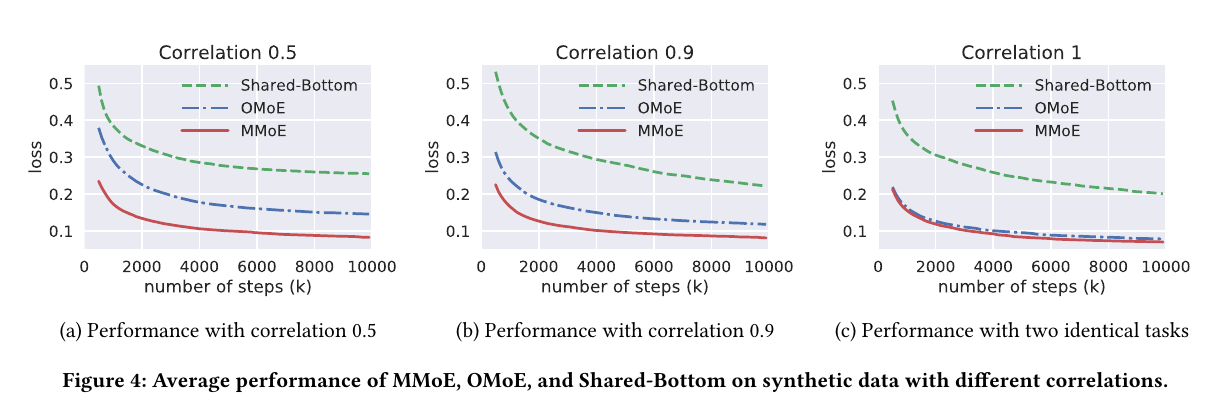• 所有的模型，任务相关性越高，表现越好
• 随着任务相关性的差别造成的MMoE model表现的差异要小于OMoE model以及Shared-Bottom model，这种趋势在比较MMoE model和OMoE model的时候尤为明显：
• 在极端的情况下，当两个任务完全一样的时候，MMoE和OMoE model的表现基本没有差别
• 但是随着两个任务相关性的减弱，OMoE model的表现出现明显的下降，而对MMoE model仅有微弱的影响。可见，在低相关性的情况下，gate network要因任务而异是非常重要的
• OMoE 和 MMoE model在所有情境下都优于Shared-Bottom model

#### 5.2 Trainability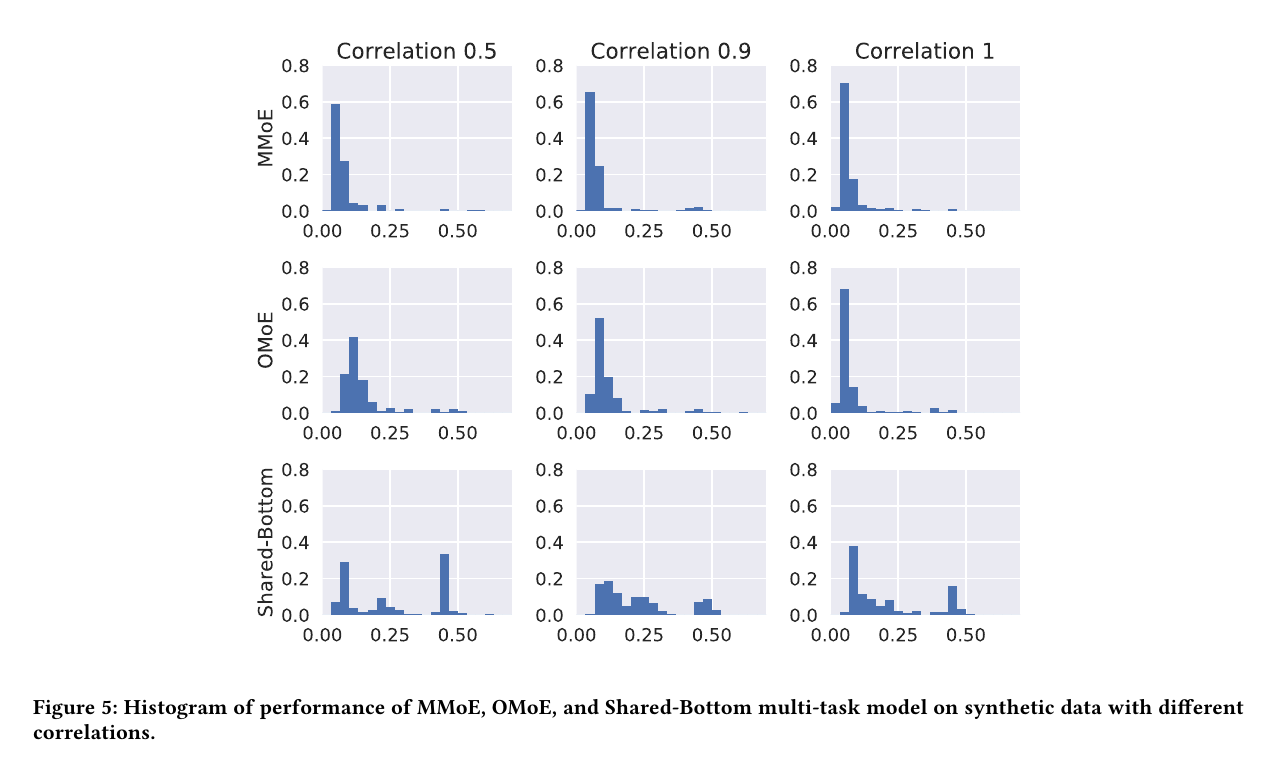• Shared-Bottom model 有更大的variances, 也就是说Shared-Bottom in general have much more poor quality local minima